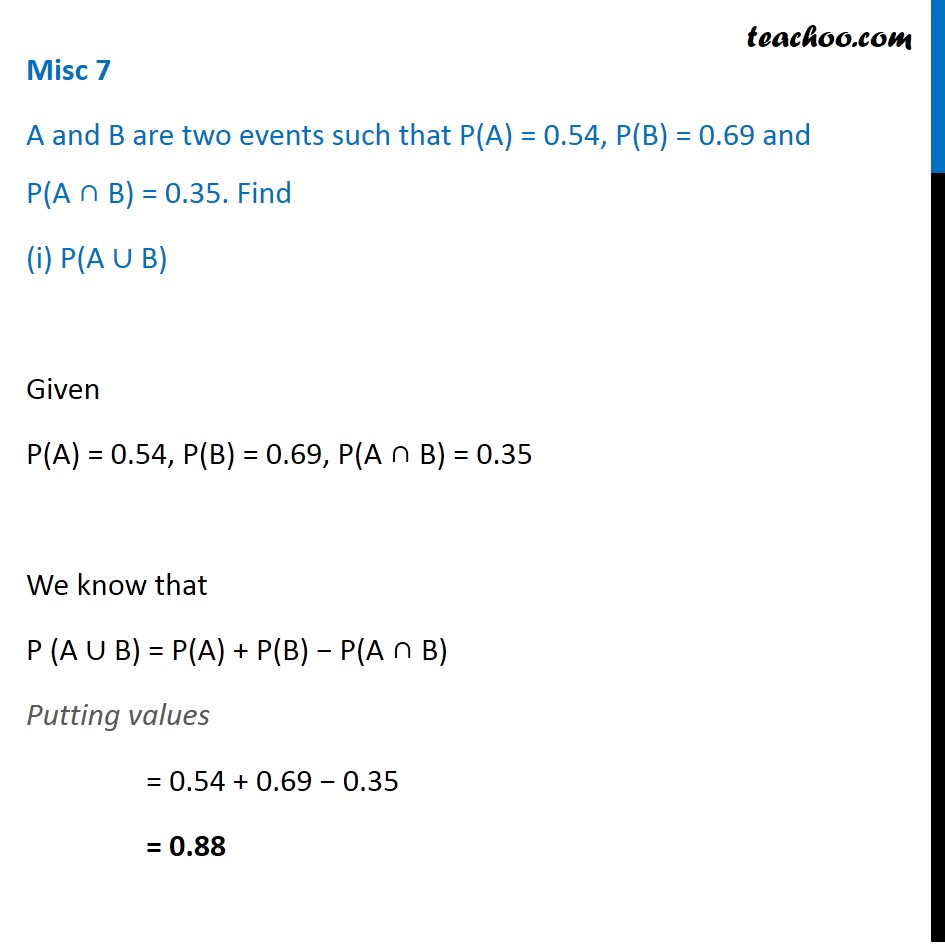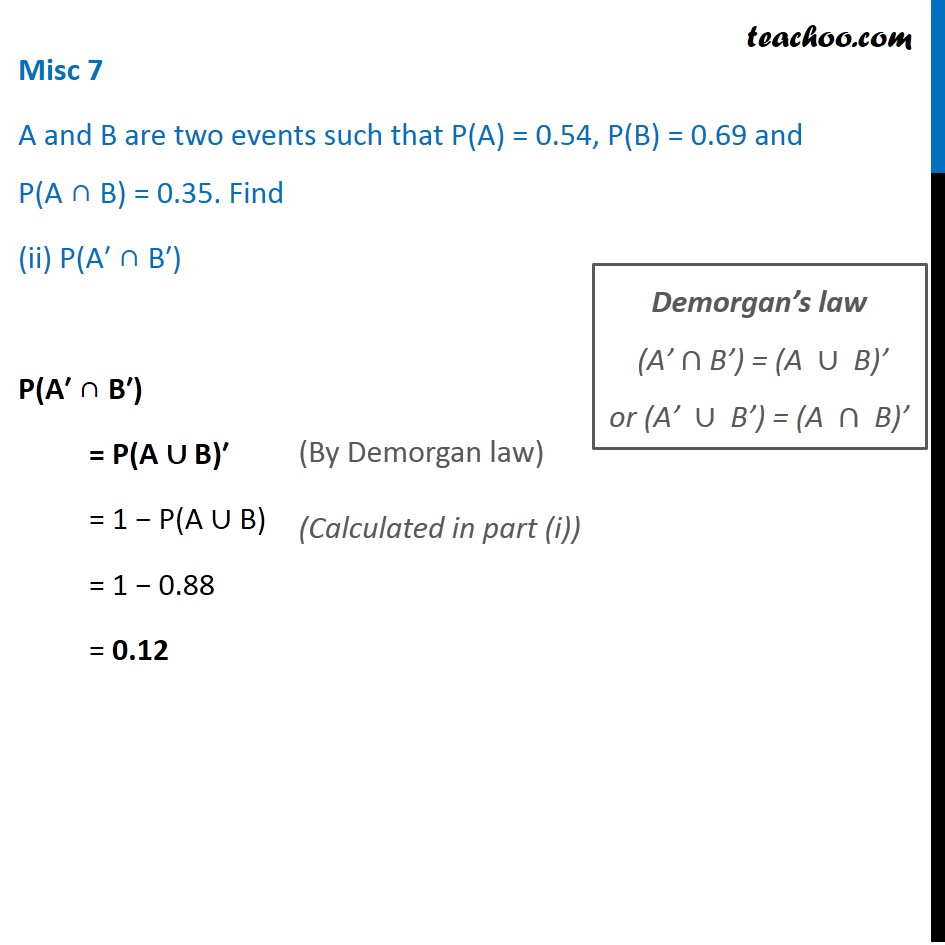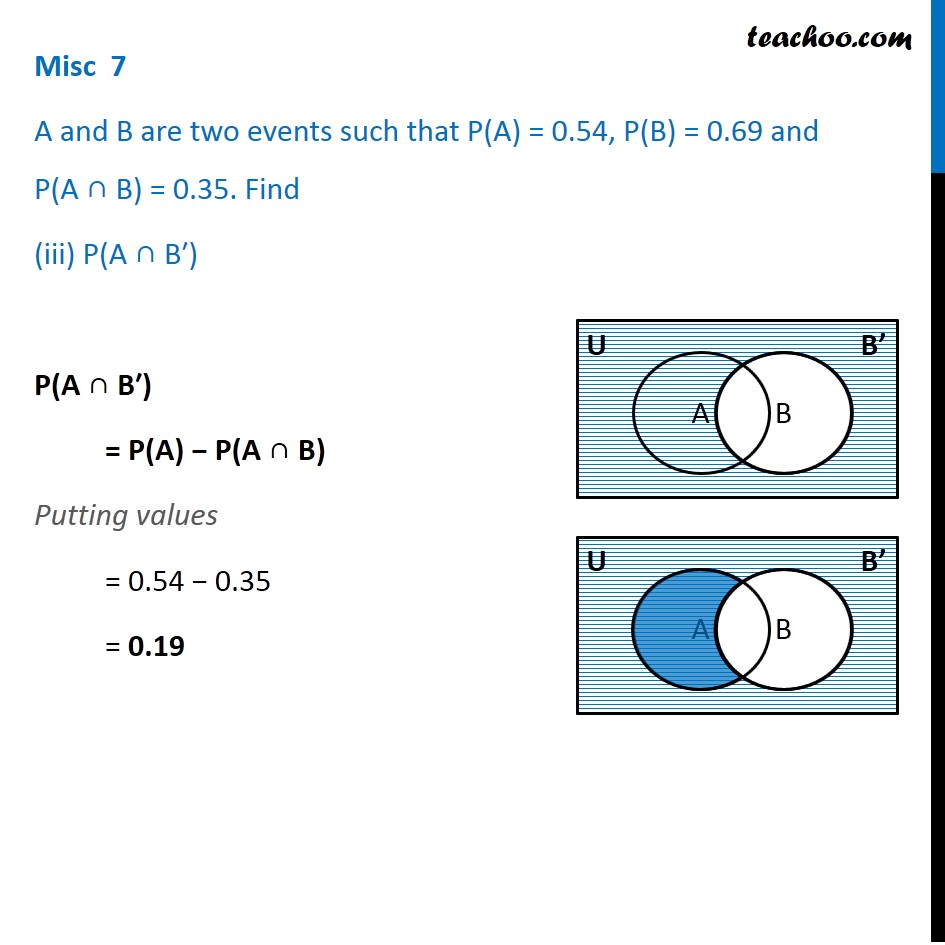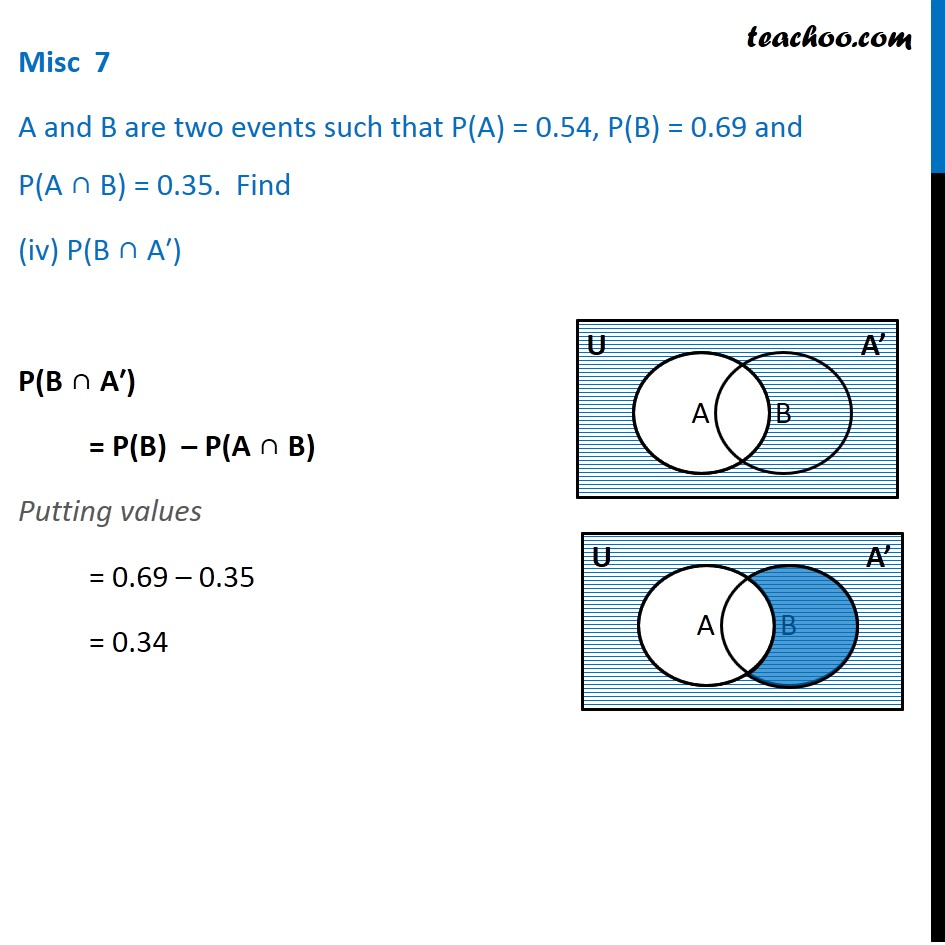Subscribe to our Youtube Channel - https://you.tube/teachoo

1. Chapter 16 Class 11 Probability
2. Serial order wise
3. Miscellaneous

Transcript

Misc 7 A and B are two events such that P(A) = 0.54, P(B) = 0.69 and P(A ∩ B) = 0.35. Find (i) P(A ∪ B) Given P(A) = 0.54, P(B) = 0.69, P(A ∩ B) = 0.35 We know that P (A ∪ B) = P(A) + P(B) − P(A ∩ B) Putting values = 0.54 + 0.69 − 0.35 = 0.88 Misc 7 A and B are two events such that P(A) = 0.54, P(B) = 0.69 and P(A ∩ B) = 0.35. Find (ii) P(A′ ∩ B′) P(A′ ∩ B′) = P(A ∪ B)′ = 1 − P(A ∪ B) = 1 − 0.88 = 0.12 (By Demorgan law) Demorgan’s law "(A’"∩"B’) = (A "∪" B)’" "or (A’ "∪" B’) = (A "∩" B)’" Misc 7 A and B are two events such that P(A) = 0.54, P(B) = 0.69 and P(A ∩ B) = 0.35. Find (iii) P(A ∩ B′) P(A ∩ B′) = P(A) − P(A ∩ B) Putting values = 0.54 − 0.35 = 0.19 Misc 7 A and B are two events such that P(A) = 0.54, P(B) = 0.69 and P(A ∩ B) = 0.35. Find (iv) P(B ∩ A′) P(B ∩ A′) = P(B) – P(A ∩ B) Putting values = 0.69 – 0.35 = 0.34

Miscellaneous